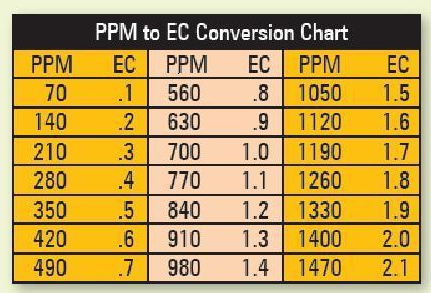# Help with reading ec meter

My ec meter gives me a 4 digit # (example: 0235). How does this number correspond to the ec number I am striving for (example: 1.0)?

sounds more like a ppm meter

1 Like

It does both, has a button to switch between functions of TDS in ppm and Conductivity in some wierd symbol in mill somethings per millamole or some craziness. Anyway, I can use the TDS, oretty straight forward, but I am confused in reading the ec.

ppm is just as effective as EC and simpler to work with for most more precise

2 Likes

Good information. THANKS!

TDS meters actually use EC to calculate PPM’s. Problem is, not every TDS meter uses the same calculation.

Here is a chart to help convert if you need it. There are many out there.2 Likes

Agreed, too high for EC, sounds like PPM unit of measure to me as well.

@Dank Sounds like your EC meter reads in microsiemens [µS]. EC recommendations are given in terms of milisiemans [mS]. A microsiemen is 1000x smaller than a milisiemen. Therefore, 2200 [µS] = 2.2 [mS].

In other words, just move the decimal three places to the left. Since your meter is 4 digits, this means you can simply pretend there is a decimal point after the first number.9 Likes

@GrowManFitz, so if my ec meter reads 0235, that would be an ec of 0.235?

1 Like

Assuming your meter reads in terms of microsiemens, that would be correct. Is there a little symbol after the reading that looks like [µS]?

2 Likes

Yes, it does.

I’ll post a pic of my meter later. Mine also reads in terms of microsiemens [µS].

Then yes 0235 would be an EC of 0.235.1 Like

That is very yelpful! Thanks @GrowManFitz!

@Dank No problem! I had the same question when I first got my meter. Now you’ll be able to help someone else. That’s what makes this forum great!

5 Likes